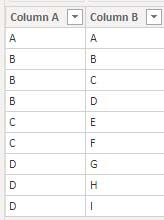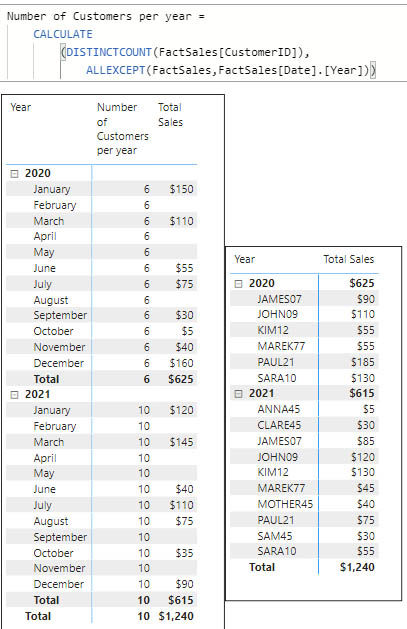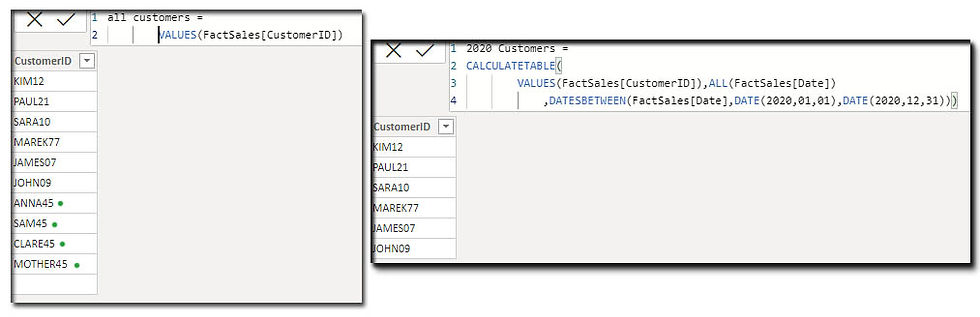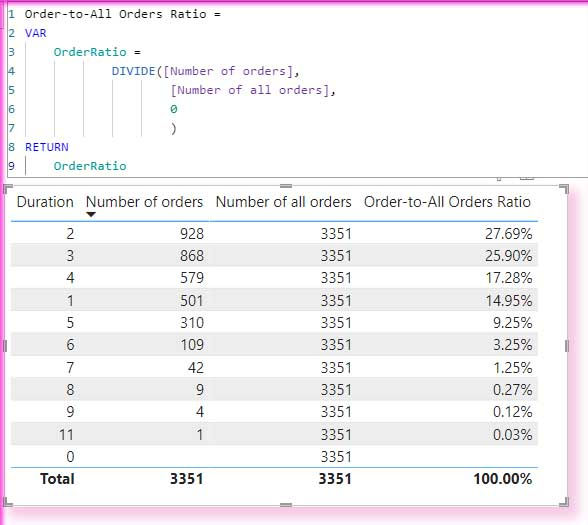top of page
Search
• Marek Vavrovic

# Mixed Scenarios.

Updated: May 5, 2022

Count values in one column based on another column in DAX

I want to create a calculated column which will contain the sum of occurrences of values in Column A based on the values in Column B.Example 1

using EARLIER (as a new column)Example 2

using FILTER(ALL ()(as a new column)Example 3

Earlier in measure

Earlier function needs 2 nested row contexts to work with. You need to get this function 2 tables. It is not working just with 1 table in a measure.

Occurence =

VAR

RowCount = Sumx(CategoryData,

COUNTROWS(FILTER(ALL(CategoryData),

EARLIER(CategoryData[Subcategory])=CategoryData[Category])))

RETURN

RowCountCalculate SUM for a single item

Salary for Maria = CALCULATE( SUM(DimCustomer[Salary]), FILTER(VALUES(DimCustomer),DimCustomer[Customer]="Maria"))## Partitioning data by month

Calculating average per month.Step A

calculating sum per month.

I have partitioned data by Year_month and using ALLEXCEPT(FactSales,FactSales[Year_month])) to calculate sum per month.

Step B

calculating number of transaction per month. Partitioning data by Year_month, using ALLEXCEPT(FactSales,FactSales[Year_month])), the same logic as in step A.Step C

Calculating average by dividing sum of the group by the number of its data points. Data on the chart are sorted by the Index column, which is in the tooltip.

MEAN By Year_Month =

VAR s =

CALCULATE (

SUM ( FactSales[Sales] ),

ALLEXCEPT ( FactSales, FactSales[Year_month] )

)

VAR d =

CALCULATE (

COUNTROWS ( FactSales ),

ALLEXCEPT ( FactSales, FactSales[Year_month] )

)

RETURN

DIVIDE ( s, d, 0 )## Calculate running total just for the first 15 days

FIRST 15 DAYS CUM_SALE =

VAR Period =

DATESBETWEEN ( tblSales[Date], DATE (2021, 01, 01), DATE (2021, 1, 15))

VAR _RESULT =

CALCULATE (

SUM ( tblSales[Sales] ),

DATESYTD ( tblSales[Date], "2021-01-15"),

Period

)

RETURN _RESULT## Calculate running total for selected days.

Step 1

create measure that calculates the running total

Total Sales = CALCULATE(SUM(tblSales[Sales]),DATESMTD(tblSales[Date]))

Step 2

create a new table with selected dates

HelpTable =

CALCULATETABLE (

ADDCOLUMNS (VALUES ( tblSales[Date] ), "Total sales", [Total Sales] ),

tblSales[Date] >= DATE (2021, 01, 03)

&& tblSales[Date] <= DATE (2021, 01, 10))Step 3

Create a relationship between tblSales and HelpTable.

Step 4

Create a measure with the SUM() function.## New Customer Analysis

This measure calculates the total number of the customers per year. In 2020 there was 6 distinct customer, in 2021, 10 distinct customer. I want to find out the number of the new customers in 2021, means a new customer is every customer having no record in 2020

Number of Customers per year =

CALCULATE (

DISTINCTCOUNT ( FactSales[CustomerID] ),

ALLEXCEPT ( FactSales, FactSales[Date].[Year] ))Step 1

I want to create a measure which will contain these two tables as a virtual tables.

<all customers> table returns all the customers from 2020-2021.

<2020 Customers> table returns just the customers who have some record in 2021.

Then I use the EXECPT() function to retrieve just those customers who occur only in 2021 table.Step 2

NEW CUSTOMERS =

VAR ALL_CUSTOMER = VALUES ( FactSales[CustomerID] )

VAR CUSTOMER_PRIOR_YEAR =

CALCULATETABLE (

VALUES ( FactSales[CustomerID] ),

ALL ( FactSales[Date] ),

DATESBETWEEN ( FactSales[Date], DATE (2020, 01, 01), DATE (2020, 12, 31))

)

VAR RESULT =

COUNTROWS (EXCEPT (ALL_CUSTOMER, CUSTOMER_PRIOR_YEAR))

RETURN RESULTthis is how the table looks like when is filtered by 2021 year.## Cumulative percentage from delivery days

Sample data from Contoso database:Calculating an average of delivery days.Calculating number of distinct orders per delivery daysCalculating the grand total of all distinct order.Calculating a ratio of number deliveries per delivery duration to its grand total.

85% of all deliveries are delivered within the first 4 days. If I order an item on Wednesday morning, on Wednesday or Thursday night will be processed and there is an 85% probability that on Friday my item will be delivered, speaking theoretically.Calculating a cumulative percentage of all orders delivered up to 4-day. This measure shows a proportion of all orders delivered within 4 days cumulatively.Creating a disconnected Days table. This table will be used in a matrix visual in columns. I going to iterate over these values, use this table to calculate the cumulative percentage. SELECTCOLUMNS function takes a table and columns from an existing table. It contains an option to specify our own names (you can rename the original column names) for the newly created / extracted table.this shows how to use that table with Up-to-4-day Delivery measure, put it into columns in matrix.The next step is to grab the value from Days table and use it in a measure.

We can use SELECTEDVALUE function which supplies the actual values from Days table.

The result we are looking at is displaying the values cumulatively. If we look at value 4 for Australia in the first row, we can conclude that up to 4 days 84.3% of deliveries are completed.

Days 6 and 7 are missing because I did not add these values to my table. But you can of course.As you can see, we got repeating 100% values we must take care of. And, as you can see CurrentDaysValue - 1 did not fix this problem.The final measure:

Cumulative Percentage =

VAR

CurrentDaysValue = SELECTEDVALUE(Days[Days])

VAR

Process =

CALCULATE([Order-to-All Orders Ratio],

FactSales[Duration] <= CurrentDaysValue)

VAR

MaxCurrentDay = CALCULATE(MAX(Days[Days]),Days[Days] < CurrentDaysValue)

VAR

COPYOF_Process =

CALCULATE([Order-to-All Orders Ratio],

FactSales[Duration] <= MaxCurrentDay)

RETURN

IF(COPYOF_Process < 1 , Process)Adding a conditional formating for values

CumulativePercFormatting =

var TargetTable =

CALCULATE( ROUND( [Cumulative Perc], 4),

REMOVEFILTERS(), --remove filters.

VALUES(DeliveryTime[Days])) --restore filters for columns in Matrix Vis.

var TargetValue = ROUND([Cumulative Perc],4)

var Result = IF ( TargetValue < TargetTable, -1, +1 )

return

Result

Values below the grand total average are formatted red.## Grouping a table using SUMMARIZECOLUMN function

Sample data: Using tables Order and OrderDetails from Northwind database. Tables are joined into one table. I've added a Year column for groupping.I want to create a forecast for 1998 year. This year finish at 06/05/1998. Each year has 4 quarters. I calculate the sum of 1998 and substract the value which is over Q1. I will get the net value for Q1 and then I multiply that value by 3 assuming all 4 quarters will have the same total.Groupping the columns

OrdersForCAGR =

var TargetTable =

SUMMARIZECOLUMNS(Orders[Year], "Total",SUM(Orders[Total Sales])),

"Quarterly Coefficient", CALCULATE(1/(4/12))),

"Sales Total", IF(Orders[Year] = 1998,

CALCULATE([Sales] * (1/(4/12))) - [Sales 1998 over Q1],

CALCULATE([Sales])))

return

TargetTable

If condition checks if Year = 1998. If returns TRUE, sum for 1998 will be multiplied by 3 and I substract the sum which is after 1. April. Values for 1996 and 1997 will be just copied.Once you have it, you can use it for a simple forecast.Having a full year for 1998, can calculate CAGR. This is not very accurate with just 3 years of data.